## A batch consists of 12 defective coils and 88 good ones. Find the probability of getting two good coils when two coils are randomly selected

Question

A batch consists of 12 defective coils and 88 good ones. Find the probability of getting two good coils when two coils are randomly selected if the first selection is replaced before the second is made.

in progress 0
6 months 2021-07-27T08:02:14+00:00 1 Answers 200 views 0

## Answers ( )

0.7744 = 77.44% probability of getting two good coils when two coils are randomly selected

Step-by-step explanation:

For each coil, there are only two possible outcomes. Either it is good, or it is not. Since the coil taken is replaced, the probability of choosing a good coil on a trial is independent of any other trial, which means that the binomial probability distribution is used to solve this question.

Binomial probability distribution

The binomial probability is the probability of exactly x successes on n repeated trials, and X can only have two outcomes.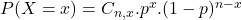In which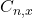is the number of different combinations of x objects from a set of n elements, given by the following formula.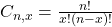And p is the probability of X happening.

88 out of 100 are good:

This means that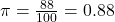Find the probability of getting two good coils when two coils are randomly selected.

This is P(X = 2) when n = 2. So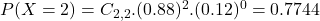0.7744 = 77.44% probability of getting two good coils when two coils are randomly selected